﻿ DIF

# DIF: differential item functioning

A couple of topics back, at "Ibreaks", an answer of No was entered when the following question appeared: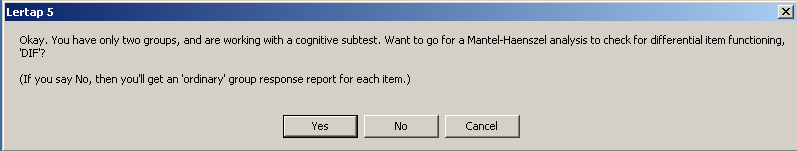Now an answer of Yes will be entered, something which prompts the Ibreaks routine to ask one more question: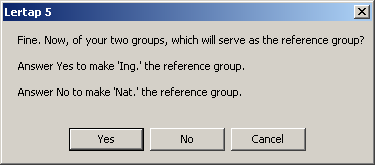For this example, an authentic one to be sure, a professionally-developed 40-item achievement test had been used for years as an important assessment tool in science education.  It had been presented in the country's native language, that is, the one most used in the general population.  However, a push to promote the wider use of English, a strong, widely used second language, eventually resulted in high school science and mathematics instruction switching to English.  Selected assessment instruments were carefully translated to English; cycles of forward and back translations were used to control the process, and, after trials stretching over three years, many of these instruments came into main-stream application.

In this example the Nat. group served as the reference group.  The native-language version of the test was seen as the "gold standard"; some educators thought that the switch to English was disadvantageous -- the focus was on students sitting the English version of the test, and the question was: was it a fair test?  Was there any evidence to suggest that the English version of the test worked against students, putting them at a disadvantage when compared to those who might still get to sit the native-language version of the test?

So it was that the answer to this Ibreaks question was No.  This served to define the Nat. group as the reference group.  The Ing. group became the "focal" group.

Once Ibreaks has an answer, it starts to churn out results.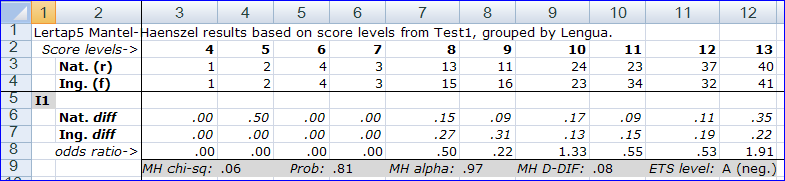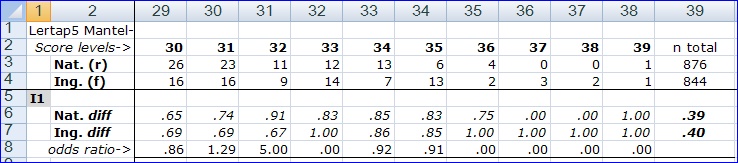When a DIF analysis has been requested, Lertap's Ibreaks routine creates two new reports, or worksheets.  The snapshots above are from one of these, the "IbrakesMH" report.  MH stands for Mantel-Haenszel, the method Ibreaks uses for its DIF analysis.

The Score levels row starts at the lowest test score found, 4, and continues, in steps of 1 (one) to the highest score, which in this case was 39.  These tables can become quite wide.  The screen snapshots above have captured the first ten score levels, and the last ten.

Rows 3 and 4 give the number of students in each group at each score level.  These numbers start to become relatively "substantial" at score level 8 (column 7), with 13 students in the (r)eference group, and 15 in the (f)ocal group.

The two diff rows give the proportion of students in each group who answered the item correctly.  The odds ratio is a relative measure of how likely it is that a student in the reference group will get an item correct when compared to a student in the focal group.  Greater than one, and the odds favour members of the reference group as being more likely to return the right answer.  Less than one, and the focal group has the advantage.  Equal to one, and it's even-steven.

The final column of the table gives the number of students in each group (876 and 844), and then, for each item, the proportion of correct responses for each group, over all score levels.  Because this version of Lertap Ibreaks looks only at items which have been scored on a right / wrong basis, these proportions are equivalent to classical item difficulty figures.  Thus, for item I1, the difficulty was .39 in the reference group, and .40 in the focal group.

Row 9 above has MH statistics for I1. MH alpha is the "common odds ratio", a figure derived by forming an average of the odds ratios over all score levels, weighted by the number in each group at each score level.  In this example, an MH alpha of .97 indicates that the odds favour the focal group, but, since a value of 1.00 indicates equal odds, it's not a big favour.

MH chi-sq. is used to test the hypothesis that MH alpha is equal to one in the population from which the two samples of students have been drawn. Prob: is used to judge the statistical significance of MH chi-sq.  We'd generally say that MH chi-sq. is statistically significant when Prob: is equal to or less than .05, and, this being the case, we would be tempted to say that MH alpha is, in fact, not equal to one; in turn, if this is indeed so, then we have evidence suggesting that group membership makes a difference: the chances of us observing a correct answer from a student might be said to depend on which group s/he is a member of.

Now, if it can be shown that the students in each group are of equal proficiency, or ability, then what might it be which would make it more likely for one of the groups to get an item correct?  Perhaps a difference in the two versions of the item?  Perhaps we have evidence of DIF, differential item functioning.  This is often unwanted.  If the objective is to have a "fair" test, test developers will generally weed out items which have such behavior (see tidbits references below; Angoff has a discussion of when DIF might be tolerated, even expected).

MH D-DIF is a statistic which results from converting MH alpha to a different scale: MH D-DIF = -2.35 times the natural logarithm of MH alpha.  The D in D-DIF stands for delta.  The delta metric is used by ETS, the Educational Testing Service, to express item difficulty.

Items with positive MH D-DIF favour the focal group, negative MH D-DIF favours the reference group.

The ETS level for an item will be A, B, or C.  It will be A if MH D-DIF is between negative one and plus one (-1.00 < MH D-DIF < +1.00), or if Prob. is greater than .05 (MH chi-sq. is not statistically significant).  A-level items are said to indicate negligible DIF; the IbreaksMH tables show this as A (neg.). Note: yes, it is possible to have a statistically-significant MH chi-sq., but still have the item falling into the ETS A level if the magnitude of MH D-Dif is less than 1.00.

An item with substantial DIF, the C level on the ETS "scale", has an MH D-DIF value with a magnitude of at least 1.5 (that is, at or above 1.5, or at or below -1.5), with MH D-DIF significantly greater than 1.0 in magnitude.  These items are denoted in IbreaksMH tables as C (large). Note: not shown in the examples on this page is another statistic which appears to the right of the ETS level, "s.e.", the standard error of MH D-DIF. (A concise reference for the calculations used in this part of Lertap is Michaelides (2008); see Dorands & Kulick (2006) for a practical application of MH statistics and discussion of the ETS "scale" -- see "Related tidbits" below.)

Bet you can't guess what an ETS B-level item is?  It's one that is not in one of the other two levels.  It exhibits some DIF, moderate DIF is the correct ETS term, and IbreaksMH tables show this as B (mod.).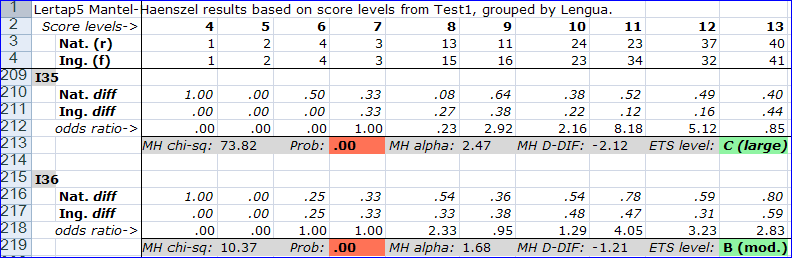Here we have examples of two items which favour the reference group, Nat.  The MH D-DIF figure for both of these items, I35 and I36, is high. Whenever MH D-DIF is outside of the range +1 to -1, it can be useful to get a picture which encapsulates some of the information in an IbreaksMH table -- the next topic has an example.

Plots.  Above it says that asking for a DIF analysis gives "two new reports".  IbreaksMH is one.  The other is a version of the usual Ibreaks reports; this time its charts will come with DIF data included: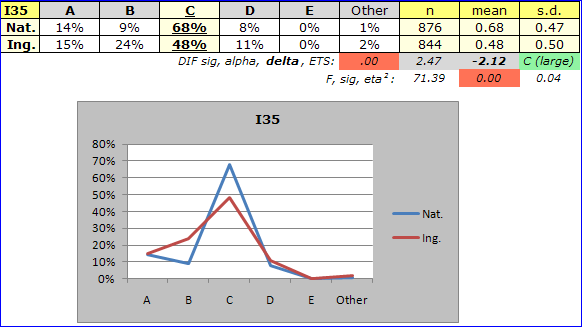The line of DIF stats has four fields imported from IbreaksMH tables: Prob. is here called sig, MH alpha is just called alpha, MH D-DIF becomes delta, and ETS is unaltered.

More plots.  IbreaksMH tables given ample opportunity to make additional Excel charts.  Examples are given in the next topic, Enhance an Ibreaks MH chart.

Related tidbits:

There's a paper on the Lertap website with more about DIF, especially as implemented in Ibreaks.  Please see: http://www.lertap5.com/Documentation/GimmeABreak1.pdf.

One of our videos shows how to use Lertap 5's DIF features -- see Video (5) at this webpage.

For DIF references, see Angoff (1993), Camilli & Shepard (1994),  Clauser & Mazor (1998), Dorans & Holland (1993), Dorans & Kulick (1986), Dorans & Kulick (2006), Michaelides (2008), Zieky (2003), and Zwick (2012). (Refer to this list of references.) Note: DIF is sometimes also referred to as "item bias", now regarded by many as an outmoded term.